PROC ACECLUS Statement
PROC ACECLUS PROPORTION=p | THRESHOLD=t <options> ;

The PROC ACECLUS statement starts the ACECLUS procedure. The options available with the PROC ACECLUS statement are summarized in Table 23.2 and discussed in the following sections. Note that, if you specify the METHOD=COUNT option, you must specify either the PROPORTION= or the MPAIRS= option. Otherwise, you must specify either the PROPORTION= or THRESHOLD= option.

Table 23.2 Summary of PROC ACECLUS Statement Options

Options

Description

Specify clustering options

Specifies the clustering method

Specifies number of pairs for estimating within-cluster covariance (when you specify the option METHOD=COUNT)

Specifies proportion of pairs for estimating within-cluster covariance

Specifies the threshold for including pairs in the estimation of the within-cluster covariance

Specify input and output data sets

Specifies input data set name

Specifies output data set name

Specifies output data set name containing various statistics

Specify iteration options

Uses absolute instead of relative threshold

Specifies convergence criterion

Specifies initial estimate of within-cluster covariance matrix

Specifies maximum number of iterations

Specifies metric in which computations are performed

Specifies singularity criterion

Specify canonical analysis options

Specifies number of canonical variables

Specifies prefix for naming canonical variables

Control displayed output

Suppresses the display of the output

Produces PP-plot of distances between pairs from last iteration

Produces QQ-plot of power transformation of distances between pairs from last iteration

Omits all output except for iteration history and eigenvalue table

The following list provides details about the options.

ABSOLUTE

causes the THRESHOLD= value or the threshold computed from the PROPORTION= option to be treated absolutely rather than relative to the root mean square distance between observations. Use the ABSOLUTE option only when you are confident that the initial estimate of the within-cluster covariance matrix is close to the final estimate, such as when the INITIAL= option specifies a data set created by a previous execution of PROC ACECLUS by using the OUTSTAT= option.

CONVERGE=c

specifies the convergence criterion. By default, CONVERGE= 0.001. Iteration stops when the convergence measure falls below the value specified by the CONVERGE= option or when the iteration limit as specified by the MAXITER= option is exceeded, whichever happens first.

DATA=SAS-data-set

specifies the SAS data set to be analyzed. By default, PROC ACECLUS uses the most recently created SAS data set.

INITIAL=name

specifies the matrix for the initial estimate of the within-cluster covariance matrix. Valid values for name are as follows:

DIAGONAL | D

uses the diagonal matrix of sample variances as the initial estimate of the within-cluster covariance matrix.

FULL | F

uses the total-sample covariance matrix as the initial estimate of the within-cluster covariance matrix.

IDENTITY | I

uses the identity matrix as the initial estimate of the within-cluster covariance matrix.

INPUT=SAS-data-set

specifies a SAS data set from which to obtain the initial estimate of the within-cluster covariance matrix. The data set can be TYPE=CORR, COV, UCORR, UCOV, SSCP, or ACE, or it can be an ordinary SAS data set. See Appendix A, Special SAS Data Sets, for descriptions of CORR, COV, UCORR, UCOV, and SSCP data sets. See the section Output Data Sets for a description of ACE data sets.

If you do not specify the INITIAL= option, the default is the matrix specified by the METRIC= option. If neither the INITIAL= nor the METRIC= option is specified, INITIAL=FULL is used if there are enough observations to obtain a nonsingular total-sample covariance matrix; otherwise, INITIAL=DIAGONAL is used.

MAXITER=n

specifies the maximum number of iterations. By default, MAXITER=10.

METHOD=COUNT | C | THRESHOLD | T

specifies the clustering method. The METHOD=THRESHOLD option requests a method (also the default) that uses all pairs closer than a given cutoff value to form the estimate at each iteration. The METHOD=COUNT option requests a method that uses a number of pairs, m, with the smallest distances to form the estimate at each iteration.

METRIC=name

specifies the metric in which the computations are performed, implies the default value for the INITIAL= option, and specifies the matrix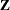used in the formula for the convergence measure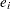and for checking singularity of the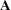matrix. Valid values for name are as follows:

DIAGONAL | D

uses the diagonal matrix of sample variances diag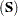and sets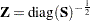, where the superscript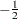indicates an inverse factor.

FULL | F

uses the total-sample covariance matrix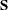and sets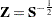.

IDENTITY | I

uses the identity matrixand sets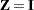.

If you do not specify the METRIC= option, METRIC=FULL is used if there are enough observations to obtain a nonsingular total-sample covariance matrix; otherwise, METRIC=DIAGONAL is used.

The option METRIC= is rather technical. It affects the computations in a variety of ways, but for well-conditioned data the effects are subtle. For most data sets, the METRIC= option is not needed.

MPAIRS=m

specifies the number of pairs to be included in the estimation of the within-cluster covariance matrix when METHOD=COUNT is requested. The values of m must be greater than 0 but less than or equal tototfq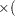totfq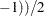, where totfq is the sum of nonmissing frequencies specified in the FREQ statement. If there is no FREQ statement, totfq equals the number of total nonmissing observations.

N=n

specifies the number of canonical variables to be computed. The default is the number of variables analyzed. N=0 suppresses the canonical analysis.

NOPRINT

suppresses the display of all output. Note that this option temporarily disables the Output Delivery System (ODS). For more information, see Chapter 20, Using the Output Delivery System.

OUT=SAS-data-set

creates an output SAS data set that contains all the original data as well as the canonical variables having an estimated within-cluster covariance matrix equal to the identity matrix. If you want to create a permanent SAS data set, you must specify a two-level name. See SAS Language Reference: Concepts for more information about permanent SAS data sets.

OUTSTAT=SAS-data-set

specifies a TYPE=ACE output SAS data set that contains means, standard deviations, number of observations, covariances, estimated within-cluster covariances, eigenvalues, and canonical coefficients. If you want to create a permanent SAS data set, you must specify a two-level name. See SAS Language Reference: Concepts for more information about permanent SAS data sets.

PROPORTION=p
PERCENT=p
P=p

specifies the percentage of pairs to be included in the estimation of the within-cluster covariance matrix. The value of p must be greater than 0. If p is greater than or equal to 1, it is interpreted as a percentage and divided by 100; PROPORTION=0.02 and PROPORTION=2 are equivalent. When you specify METHOD=THRESHOLD, a threshold value is computed from the PROPORTION= option under the assumption that the observations are sampled from a multivariate normal distribution.

When you specify METHOD=COUNT, the number of pairs, m, is computed from PROPORTION=p as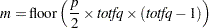where totfq is the number of total nonmissing observations.

PP

produces a PP probability plot of distances between pairs of observations computed in the last iteration.

PREFIX=name

specifies a prefix for naming the canonical variables. By default the names are Can1, Can2, ..., CANN. If you specify PREFIX=ABC, the variables are named ABC1, ABC2, ABC3, and so on. The number of characters in the prefix plus the number of digits required to designate the variables should not exceed the name length defined by the VALIDVARNAME= system option. For more information about the VALIDVARNAME= system option, see SAS System Options: Reference.

QQ

produces a QQ probability plot of a power transformation of the distances between pairs of observations computed in the last iteration. Caution: The QQ plot can require an enormous amount of computer time.

SHORT

omits all items from the standard output except for the iteration history and the eigenvalue table.

SINGULAR=g
SING=g

specifies a singularity criterion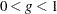for the total-sample covariance matrixand the approximate within-cluster covariance estimate. The default is SINGULAR=1E4.

THRESHOLD=t
T=t

specifies the threshold for including pairs of observations in the estimation of the within-cluster covariance matrix. A pair of observations is included if the Euclidean distance between them is less than or equal to t times the root mean square distance computed over all pairs of observations.